# ML | Intercluster and Intracluster Distance

Cluster Analysis –
The aim of the clustering process is to discover overall distribution patterns and interesting correlations among the data attributes. It is the task of grouping a set of objects in such a way that objects in the same group are more similar to each other than to those in other groups. Cluster analysis itself is not one specific algorithm, but the general task to be solved. It can be achieved by various algorithms that differ significantly in their understanding of what constitutes a cluster and how to efficiently find them. Popular notions of clusters include groups with small distances between cluster members, dense areas of the data space, intervals or particular statistical distributions.

Here, we will discuss about the distance between the objects of the different clusters and the objects of the same clusters. We have two type of distance – Intercluster Distance and Intracluster Distance.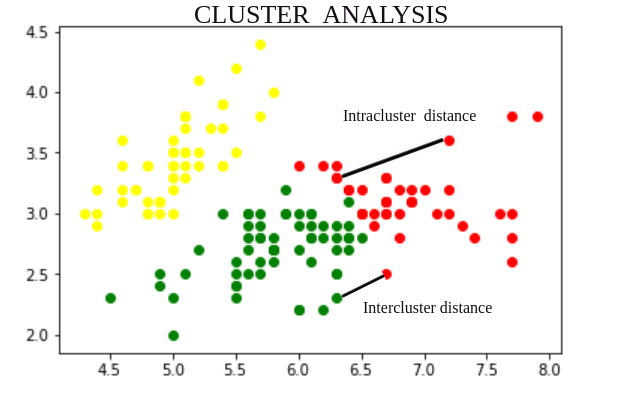Let S and T are clusters formed using partition U. `d(x, y)` is the distance between two objects x and y belonging to S and T respectively. `d(x, y)` is calculated using well-known distance calculating methods such as Euclidean, Manhattan and Chebychev. |S| and |T| are the number of objects in clusters S and T respectively.

### Intercuster Distance:

Intercluster distance is the distance between two objects belonging to two different clusters. It is of 5 types –

1. Single Linkage Distance : The single linkage distance is the closest distance between two objects belonging to two different clusters defined as –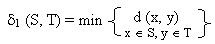2. Complete Linkage Distance : The complete linkage distance is the distance between two most remote objects belonging to two different clusters defined as –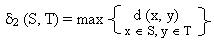3. Average Linkage Distance : The average linkage distance is the average distance between all the objects belonging to two different clusters defined as –4. Centroid Linkage Distance : The centroid linkage distance is the distance between the centers vs and vt of two clusters S and T respectively, defined as –where,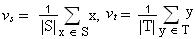5. Average Centroid Linkage Distance : The average centroid linkage distance is the distance between the center of a cluster and all the objects belonging to a different cluster, defined as –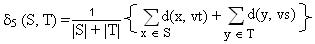### Intracuster Distance:

Intracluster distance is the distance between two objects belonging to same cluster. It is of 3 types –

1. Complete Diameter Distance : The complete diameter distance is the distance between two most remote objects belonging to the same cluster defined as –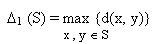2. Average Diameter Distance : The average diameter distance is the average distance between all the objects belonging to the same cluster defined as –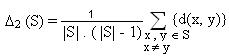3. Centroid Diameter Distance : The centroid diameter distance is double average distance between all of the objects and the cluster center of s defined as –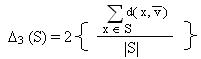where,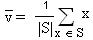Note:
If a clustering algorithm makes clusters so that the Intercluster distance between different clusters is more and Intracluster distance of same cluster is less, then we can tell that it is a good clustering algorithm.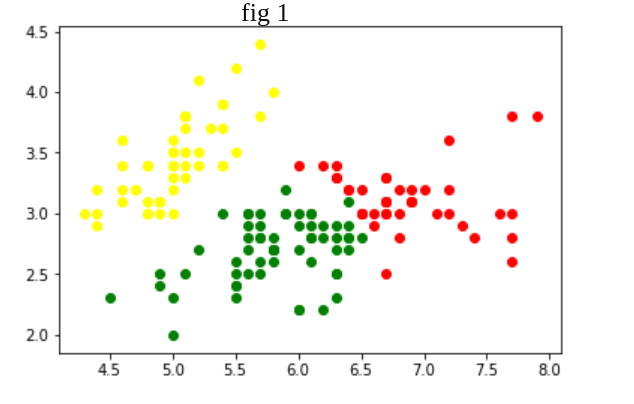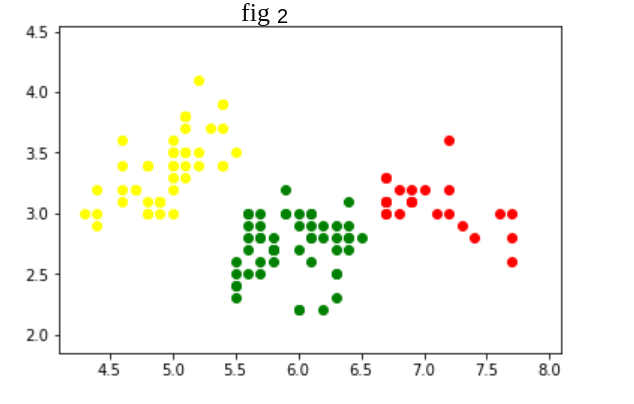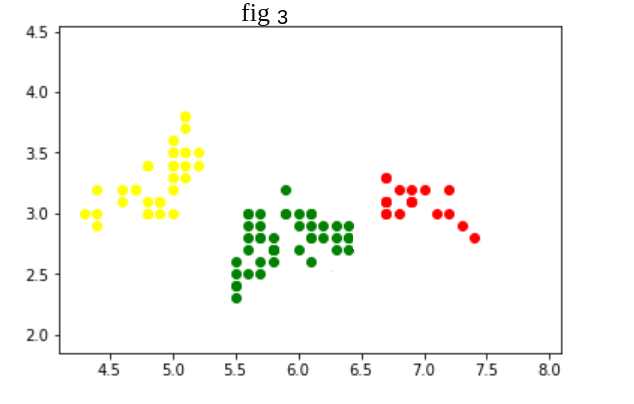Here clustering algorithm in fig 3 is better than fig 2 and fig 1 as in fig 3 Intercluster distance is more and Intracluster distance is less.

My Personal Notes arrow_drop_upCheck out this Author's contributed articles.

If you like GeeksforGeeks and would like to contribute, you can also write an article using contribute.geeksforgeeks.org or mail your article to contribute@geeksforgeeks.org. See your article appearing on the GeeksforGeeks main page and help other Geeks.

Please Improve this article if you find anything incorrect by clicking on the "Improve Article" button below.

Article Tags :
Practice Tags :

1

Please write to us at contribute@geeksforgeeks.org to report any issue with the above content.